# Planimetrics - math word problems

Study plane measurements, including angles, distances, and areas. In other words - measurement and calculation of shapes in the plane. Perimeter and area of plane shapes.

#### Number of problems found: 1947

• Chord 4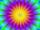I need to calculate the circumference of a circle, I know the chord length c=22 cm and the distance from the center d=29 cm chord to the circle.
• Diagonal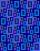Diagonal of the rectangle has length 46.4 cm. Angle between diagonal and londer sife of rectangle is 22°. Calculate area of the rectangle.
• DescribedCalculate perimeter of the circle described by a triangle with sides 478, 255, 352.
• SteepleSteeple seen from the road at an angle of 75°. When we zoom out to 25 meters, it is seen at an angle of 20°. What is high?
• Land boundaryThe land has the shape of a right triangle. The hypotenuse has a length of 30m. The circumference of the land is 72 meters. What is the length of the remaining sides of the land boundary?A domed stadium is in the shape of spherical segment with a base radius of 150 m. The dome must contain a volume of 3500000 m³. Determine the height of the dome at its centre to the nearest tenth of a meter.
• Wall and body diagonalsThe block/cuboid has dimensions a = 4cm, b = 3cm and c = 12cm. Calculates the length of the wall and body diagonals.
• Parallelogram perimeter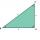The ABC triangle with sides a = 5cm, b = 3cm, c = 40mm has the center of the sides of the K,L,M. How many cm have the KBLM parallelogram perimeter?
• Surface of the cylinderCalculate the surface of the cylinder for which the shell area is Spl = 20 cm2 and the height v = 3.5 cm
• Cone roofHow many m2 of roofing is needed to cover a cone-shaped roof with a diameter of 10 m and a height of 4 m? Add an extra 4% to the overlays.
• Rectangle and circle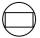The rectangle ABCD has side lengths a = 40 mm and b = 30 mm and is circumscribed by a circle k. Calculate approximately how many cm is circle long.
• Tiles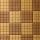How many square tiles with the content 121 cm2 has to be ordered for the paving of the square room with a side length of 2.75 meters?
• Land - isosceles trapezoidCalculate the content and perimeter of the building plot in the form of an isosceles trapezoid with bases 120m, 95m and height 50m.
• Cone - sideFind the surface area and volume of the cone if its height is 125 mm and the side length is 17 cm.
• Isosceles triangle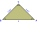In an isosceles triangle, the length of the arm and the length of the base are in ration 3 to 5. What is the length of the arm?
• Concrete pedestalThe carpenters made wooden mold on a concrete pedestal in the shape of a cube with edge 2 meter long. What is the area in which the concrete touches wooden molds? (No lid or bottom)
• Circle arcCalculate the area of the circular arc in m2 where the diameter is 290 dm and a central angle is 135°. Result round to three decimal places.
• Square ABCDConstruct a square ABCD with cente S [3,2] and the side a = 4 cm. Point A lies on the x-axis. Construct square image in the displacement given by oriented segment SS'; S` [-1 - 4].
• Isosceles trapezoidIsosceles trapezoid ABCD, AB||CD is given by |CD| = c = 12 cm, height v = 16 cm and |CAB| = 20°. Calculate area of the trapezoid.
• AnglesDetermine the interior angles of a rhombus with area 319.1 cm2 and perimeter 72 cm.

Do you have an interesting mathematical word problem that you can't solve it? Submit a math problem, and we can try to solve it.

We will send a solution to your e-mail address. Solved examples are also published here. Please enter the e-mail correctly and check whether you don't have a full mailbox.

Please do not submit problems from current active competitions such as Mathematical Olympiad, correspondence seminars etc...